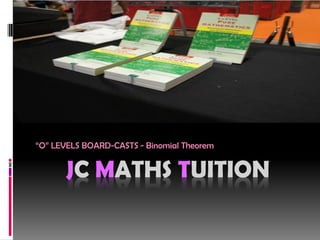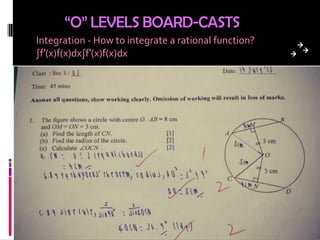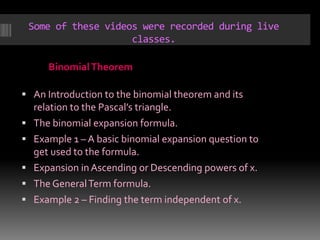Successfully reported this slideshow.

# JC Maths Tuition

1

Share×
1 of 8
1 of 8

# JC Maths Tuition

1

Share

JC Maths TUition “O” LEVELS BOARD-CASTS Integration - How to integrate a rational function and Binomial Theorem – An Introduction to the binomial theorem and its relation to the Pascal’s triangle.

JC Maths TUition “O” LEVELS BOARD-CASTS Integration - How to integrate a rational function and Binomial Theorem – An Introduction to the binomial theorem and its relation to the Pascal’s triangle.

## More Related Content

### Related Books

Free with a 14 day trial from Scribd

See all

### Related Audiobooks

Free with a 14 day trial from Scribd

See all

### JC Maths Tuition

1. 1. JC MATHS TUITION “O” LEVELS BOARD-CASTS - Binomial Theorem
2. 2. “O” LEVELS BOARD-CASTS Integration - How to integrate a rational function? ∫f′(x)f(x)dx∫f′(x)f(x)dx
3. 3. Some of these videos were recorded during live classes. BinomialTheorem  An Introduction to the binomial theorem and its relation to the Pascal’s triangle.  The binomial expansion formula.  Example 1 – A basic binomial expansion question to get used to the formula.  Expansion in Ascending or Descending powers of x.  The GeneralTerm formula.  Example 2 – Finding the term independent of x.
4. 4.  The nCr formula.  Example 3 – A binomial question that requires the use of the nCr formula.  Example 4 – Expanding 3 terms in a binomial question.  Example 5 – Challenging question with power unknown.  Example 6 – Super Challenging binomial question.  Challenging binomial theorem question.
5. 5. Is there any registration fee or any other fee? There is no registration fee but there is a \$50 material each year for JC students (starting in 2015).There is also a refundable deposit of \$100 for all students.
6. 6. No, fee is based on lessons scheduled not lessons attended. If there are 4 lessons scheduled, the fee will be based on the 4 lessons whether or not the student attends all 4 lessons. Will the fee be reduced if I were to miss a lesson?
7. 7. Call/SMS - 65 97632567 Website - www.jcmath.com.sg CONTACT